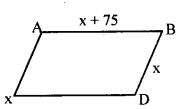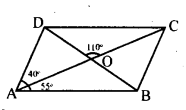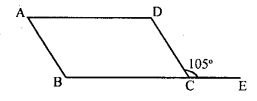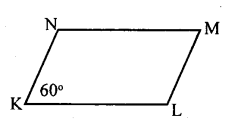# KSEEB Solutions for Class 8 Maths Chapter 15 Quadrilaterals Ex 15.3

Students can Download Maths Chapter 15 Quadrilaterals Ex 15.3 Questions and Answers, Notes Pdf, KSEEB Solutions for Class 8 Maths helps you to revise the complete Karnataka State Board Syllabus and score more marks in your examinations.

## Karnataka Board Class 8 Maths Chapter 15 Quadrilaterals Ex 15.3

Question 1.
The adjacent angles of a parallelogram are in the ratio 2 : 1. Find the measures of all the angles.
The adjacent angles are in the ratio 2 : 1.
Let the angles be 2x and x
2x + x = 180°
[adjacent angles of a parallelogram are supplementary]
3x = 180° .
x =$\frac { 180 }{ 3 }$ = 60°
2x = 2 × 60° = 120°
∴The angles of the parallelogram are 60°, 120°. 60° and 120°

Question 2.
A field is in the form of a parallelogram whose perimeter is 450m and one of its sides is larger than the other by 75m. Find the lengths of all the sides.
Let one side be x, then the other side is x + 75Perimeter = 450
x + (x + 75) + x + (x + 75) = 450
4x + 150 = 450
4x = 450 – 150
4x = 300
x = $$\frac { 300 }{ 4 }$$ = 75
x = 75m
x + 75 = 75 + 75 = 150 m
∴ The four sides are 75m, 150m, 75m, 150m.

Question 3.
In the figure ABCD is a parallelogram. The diagonals AC and BD intersect at 0; and ∠DAC = 40°, ∠CAB = 35° and ∠DOC = 110° calculate the measure ∠ABO, ∠ADC, ∠ACB and ∠CBD∠AOB = ∠DOC = 110°
[Vertically opposite angles]
In ΔAOB,
∠AOB + ∠OAB + ∠OBA = 180°
110 + 55 +∠OBA = 180°
∠OBA = 180 – 165
∠ABO = 15°
[adjacent angles of a parallelogram , are supplementary]
∠ACB = ∠DAC = 40°
∠ACB = 40°
∠ABC = ∠ ADC = 85°
[Opposite angles pf a parallelogram]
[∠ABC = ∠ABO + ∠CBO]
15 + ∠CBO = 85°
∠CBO = 85 – 15
∠CBO = 70
∴ ∠CBO = ∠CBD = 70°
∴ ∠CBD = 70°

Question 4.
In a parallelogram ABCD, the side DC is produced to E and ∠BCE = 105° calculate ∠A, ∠E, ∠C, and∠D.∠DCB + ∠DCE = 180° [Linear pair] ∠DCB + 1050 = 180°
∠DCB = 180 – 105
∠DCB = 75°
∠A = ∠DCB = 75°
∠A = ∠C = 75°
[Opposite angles of a parallelogram]
∠A +∠B = 180°
75° + ∠B = 180°
∠B = 180 + 75
∠B = 105°
∠B = ∠D = 105°
[Opposite angles of a parallelogram]

Question 5.
In a parallelogram KLMN, ∠K = 60 °. Find the measures of all the angles.